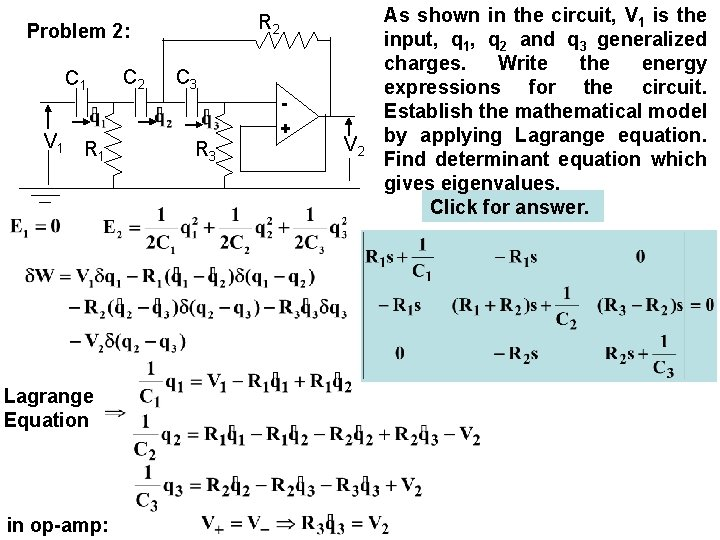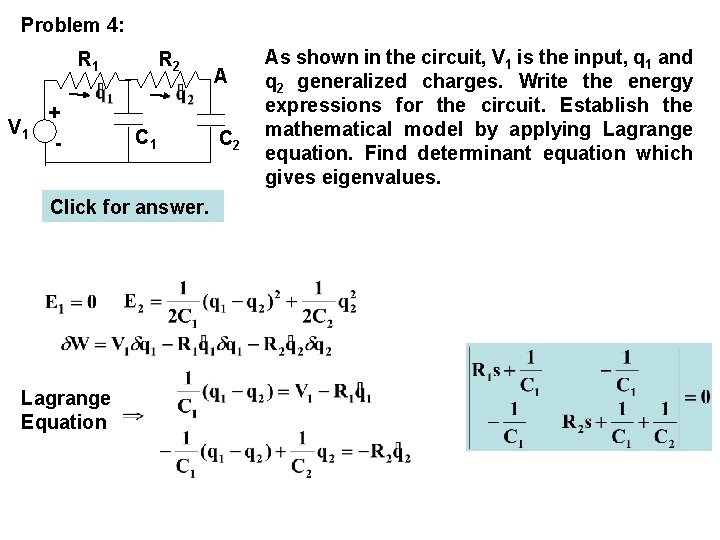# Homework 03 Electrical Circuits Problem 1 Problem 2

• Slides: 6Homework 03 Electrical Circuits: Problem 1: Problem 2: Problem 3: Problem 4: Problem 5: -+Problem 1: C L + V 1 - R As shown in the circuit, V 1 is the input, q and q 1 generalized charges. Write the energy expressions for the circuit. Establish the mathematical model by applying Lagrange equation. Find determinant equation which gives eigenvalues. Click for answer. Lagrange EquationR 2 Problem 2: C 1 V 1 R 1 Lagrange Equation in op-amp: C 2 C 3 R 3 + V 2 As shown in the circuit, V 1 is the input, q 1, q 2 and q 3 generalized charges. Write the energy expressions for the circuit. Establish the mathematical model by applying Lagrange equation. Find determinant equation which gives eigenvalues. Click for answer.Problem 3: C 1 C 2 A V 1 + - R 1 Click for answer. Lagrange Equation R 2 As shown in the circuit, V 1 is the input, q 1 and q 2 generalized charges. Write the energy expressions for the circuit. Establish the mathematical model by applying Lagrange equation. Find determinant equation which gives eigenvalues.Problem 4: R 1 V 1 + - R 2 C 1 Click for answer. Lagrange Equation A C 2 As shown in the circuit, V 1 is the input, q 1 and q 2 generalized charges. Write the energy expressions for the circuit. Establish the mathematical model by applying Lagrange equation. Find determinant equation which gives eigenvalues.C 1 Problem 5: R 1 R 2 V 1 + C 2 (Maddock, p. 499) As shown in the circuit, V 1 is the input, q 1 and q 2 generalized A charges. Write the energy expressions for the circuit. VA Establish the mathematical model by applying Lagrange equation. Find determinant equation which gives eigenvalues. Click for answer. In op-amp: Lagrange Equation